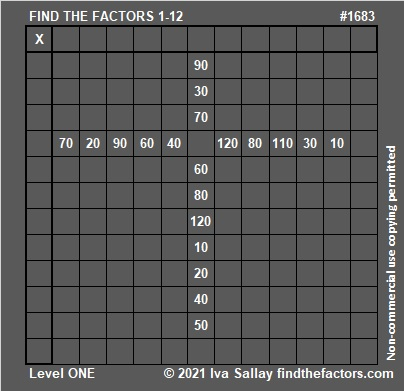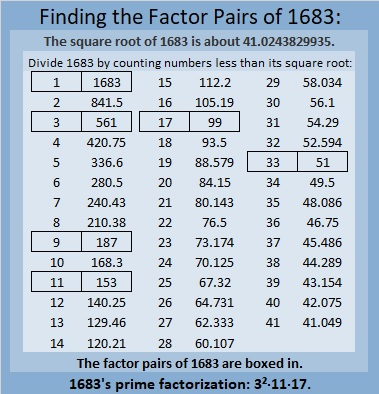# 1683 Grave Marker

Contents

### Today’s Puzzle:

It’s almost Halloween. I hope you enjoy this grave-marker puzzle. Write the numbers from 1 to 12 in both the first column and the top row so that those numbers and the given clues make a multiplication table.Here’s the same puzzle, but it won’t use up all your printer ink.### Factors of 1683:

• 1683 is a composite number.
• Prime factorization: 1683 = 3 × 3 × 11 × 17, which can be written 1683 = 3² × 11 × 17.
• 1683 has at least one exponent greater than 1 in its prime factorization so √1683 can be simplified. Taking the factor pair from the factor pair table below with the largest square number factor, we get √1683 = (√9)(√187) = 3√187.
• The exponents in the prime factorization are 2, 1, and 1. Adding one to each exponent and multiplying we get (2 + 1)(1 + 1)(1 + 1) = 3 × 2 × 2 = 12. Therefore 1683 has exactly 12 factors.
• The factors of 1683 are outlined with their factor pair partners in the graphic below.### More About the Number 1683:

1683 is the hypotenuse of a Pythagorean triple:
792-1485-1683, which is (8-15-17) times 99.

1683 is the difference of two squares in SIX different ways:
842² – 841² = 1683,
282² – 279² = 1683,
98² – 89² = 1683,
82² – 71² = 1683,
58² – 41² = 1683, and
42² – 9² = 1683.
That last one means we are 81 numbers away from the next perfect square. I also highlighted a cool-looking difference.

1680, 1681, 1682, 1683, and 1684 are the second smallest set of FIVE consecutive numbers whose square roots can be simplified.## 2 thoughts on “1683 Grave Marker”

1.Duncan Fraser

1683 is the odd leg of pythagorean triples. You have posted the m and n for the 6 triangles when you gave the difference of squares. The first pair 842 and 841 gives m minus n equals 1 and the ppt formed has a ratio of area to perimeter of 420.5(841÷2).

•ivasallay

Yes, 1683 is a side in 8 Pythagorean triples, the two I listed and the 6 you mentioned. I didn’t even think of the ratio of the area to the perimeter. Thank you very much for sharing! Did you notice my 1681st post? I quoted you a lot.

This site uses Akismet to reduce spam. Learn how your comment data is processed.